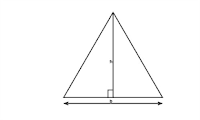### Area, Circumferance and Perimeter- Important Formulae, Tricks and Shortcuts [Quantitative Aptitude]

Here are important formulas and shortcuts to find out area, perimeter and circumference of different geometrical figures. Basic knowledge regarding some common geometrical figures like circle, rectangle, square and triangle etc is important for various competitive exams like IBPS, SBI Bank PO, assistant, SSC exams, Railway exam, UPSC exams.

### Area- Definition:

• Area is amount of space inside a boundary of 2 - D object such as circle, square, triangle etc.
• For example if there is a rectangular sheet of 1 meter length and 1 meter breadth, than its area will be 1 meter2
• If there is another sheet with length 1 meter and breadth 3 meter, so we can assume there are 3 sheets of 1 meter length and 1 meter breadth. Hence its area would be 6 meter2.

Perimeter/ Circumference- Definition:
• Perimeter / circumference is distance of the boundary of any 2 - D object.
• For example if we have a rectangular sheet of length 2 meter and breadth 1 meter, its perimeter will be total distance of the boundary of the sheet. It will be L1 + B1 + L2 + B2 = 2 + 1 + 2 + 1 = 6.

## Formulas for different figures

### 1. Rectangle:

=> Length = Area/ Breadth, { if area and breadth is given}=> Breadth = Areal / Length, { if area and length is given}

Perimeter = 2 (Length + Breadth)

=> Length = (Perimeter / 2) - Breadth,

=> Breadth = (Perimeter / 2) - Length

Related figures formulas:

Area of  parallelogram = (Base x Height)

Area of rhombus = (1/2) x (Product of diagonals)

Area of a trapezium = (1 / 2) x (sum of parallel sides) x distance between them.

### 2. Square:

Area = Length x Length

=> Length = Sq.root of (Area)

Perimeter = 4 x Length

=> Length = Perimeter / 4

### 3. Circle:Area of Circle = pi x R2    { Value of pi = 22/7, R is radius of circle}

=> R = Sq. root of (Area / pi)

Diameter = 2 x R.

=> Area = pi x  (D / 2)2r  { To find out area of circle is diameter is given}

Circumference of circle = 2 pi R

=> R = Circumference / (2 x pi)

### 4. Triangle:Area of triangle = (1/2) x Base x Height

=> Base = (2 x Areas of circle) / Height

=> Height = (2 x Areas of circle) / Base

Area of equilateral triangle = (sq.root (3) / 4 ) x (side)2

Perimeter of triangle = (Side1 + Side2 + Side3)

Perimeter of equilateral triangle = 3 x side.

### Important Points:

• Cost of painting any surface = Area of surface x cost of panting 1 square unit.
• Area of 4 walls of room with height h, width w and length l is 2(l x h + w x h).

## Want to Stay in Contact !

Get new posts and updates in your inbox. Subscribe through our Feedburner Email subscription.